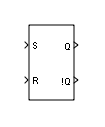# S-R Flip-Flop

Model an S-R flip-flop

• Library:
• Simulink Extras / Flip Flops

•## Description

The S-R Flip-Flop block models a simple Set-Reset flip-flop constructed using NOR gates.

The S-R Flip-Flop block has two inputs, S and R (S stands for Set and R stands for Reset) and two outputs, Q and its complement, !Q.

The truth table for the S-R Flip-Flop block follows. In this truth table, Qn-1 is the output at the previous time step.

Note

The S-R Flip-Flop block treats a nonzero input as true (`1`).

SR Q n !Q n
00 Q n-1 !Q n-1
0101
1010
1100

When S is 1 and R is 0, the flip-flop goes to the set state (Qn is 1). When R is 1 and S is 0, the flip-flop goes to the reset state (Qn is 0). When both S and R are 0, the flip-flop stays in the previous state (Qn is Qn-1).

Tip

Avoid the state where R and S are both 1. In this state, both Q and !Q are 0. This state is undefined because !Q is not the complement of Q. To handle this state, consider the J-K Flip-Flop block.

### Logic Signals as Boolean or Double Data Types

The Implement logic signals as boolean data (vs. double) configuration parameter setting affects the input and output data types of the S-R Flip-Flop block because this block is a masked subsystem that uses the Combinatorial Logic block. For more information about this configuration parameter, see Implement logic signals as Boolean data (vs. double).

## Ports

### Input

expand all

Set input, specified as a scalar, vector, or matrix.

#### Dependencies

The data types that the S-R Flip-Flop block accepts depend on the setting of the Implement logic signals as boolean data (vs. double) configuration parameter:

• `On`S-R Flip-Flop block accepts only real scalar signals of type `boolean`.

• `Off`S-R Flip-Flop block accepts real scalar signals of type `boolean` or `double` (both inputs must have the same type).

Data Types: `double` | `Boolean`

Reset input, specified as a scalar, vector, or matrix.

#### Dependencies

The data types that the S-R Flip-Flop block accepts depend on the setting of the Implement logic signals as boolean data (vs. double) configuration parameter:

• `On`S-R Flip-Flop block accepts only real scalar signals of type `boolean`

• `Off`S-R Flip-Flop block accepts real scalar signals of type `boolean` or `double` (both inputs must have the same type).

Data Types: `double` | `Boolean`

### Output

expand all

Output signal Q, with the same dimensions and data type as the input.

Data Types: `double` | `Boolean`

Output signal !Q, with the same dimensions and data type as the input.

Data Types: `double` | `Boolean`

## Parameters

expand all

Specify the initial value of the output Q. A nonzero value is treated as true (`1`).

#### Programmatic Use

 Block Parameter: `initial_condition` Type: character vector Values: scalar Default: `'0'`

## Version History

Introduced in R2008b